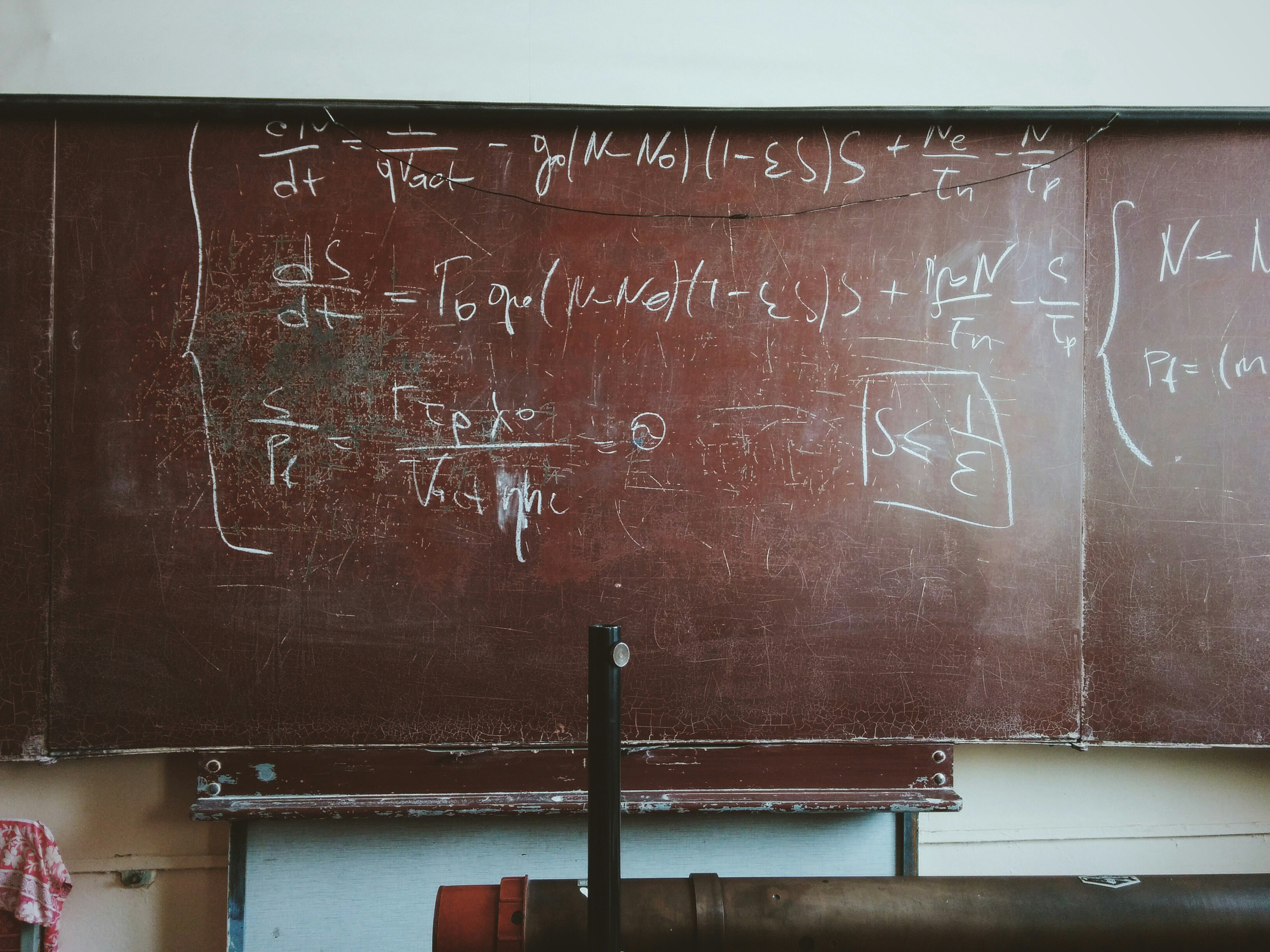# Mathematician Jokes

Matthias
in Math

An infinite number of mathematicians walk into a bar. The first one orders a beer. The second one orders half a beer. The third one orders a fourth of a beer. The bartender stops them, pours two beers and says, “You guys should know your limits.”

Anonymous

I lent a hot girl my umbrella yesterday. That takes the number of girls I’ve made wet this year to -1.

Anonymous
in Pirate

3.14% of sailors are…

π-rates.

Anonymous

What do prime numbers and stoners have in common? – The higher they are, the more spaced out they get.

Anonymous

4, 6, 8 and 9 have all been killed. – 2, 3, 5, 7 and 11 are the prime suspects.

Gage
in Programming

An infinite amount of mathematicians walked into a bar. The first ordered a pint, the second ordered a half pint, the third ordered a fourth-pint, etc. The Bartender eventually walked up and gave them two pints and said: “you mathematicians don’t know your limits.”

Mini Marshmallow
in Toilet

Did you hear about the constipated mathematician? He worked it out with a pencil

Anonymous
in Math

An chemist, a physicist, and a mathematician are stranded on an island when a can of food rolls ashore. The chemist and the physicist come up with many ingenious ways to open the can. Then suddenly the mathematician gets a bright idea: “Assume we have a can opener…”

Anonymous
in Puns

Where do mathematicians go to die?

The symmetry

Anonymous
in Math

Why don’t Romans find algebra interesting?

X is always 10.

Anonymous

If Al Gore started a math rock band it should be called Algorhythm.

Anonymous
in Religion

Why can’t atheists solve exponential equations?

Because they don’t believe in higher powers.

Anonymous
in Math

Old mathematicians never die; they just lose some of their functions.

Anonymous

The fattest knight at King Arthur’s round table was Sir Cumeference. He acquired his size from too much pi

Anonymous

A mathematician stumbles home drunk at 3 a.m. and his wife is livid. “You swore that you’d be home by 11:45!” “No,” slurs the mathematician, “I said I’d be home by a quarter of 12.”

Anonymous

I had a conversation with a Möbius strip. – It was one-sided.

Anonymous

How does a mathematician get tan? – sin/cos.

An infinite number of mathematicians walk into a bar. The first one orders a beer. The second orders half a beer. The third orders a third of a beer. The bartender bellows, “Get the hell out of here, are you trying to ruin me?”

Anonymous

What does the B in Benoît B. Mandelbrot stand for?

Benoît B. Mandelbrot

Anonymous

Hi boyyyy